# GSEB Solutions Class 6 Maths Chapter 10 Mensuration Ex 10.1

Gujarat Board GSEB Textbook Solutions Class 6 Maths Chapter 10 Mensuration Ex 10.1 Textbook Questions and Answers.

## Gujarat Board Textbook Solutions Class 6 Maths Chapter 10 Mensuration Ex 10.1

Question 1.
Find the perimeter of each of the following figures: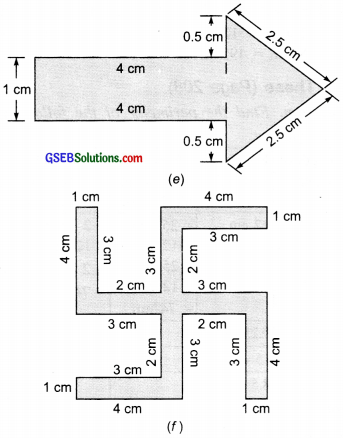Solution:
(a) Perimeter of the given figure = sum of the sides
= 4 cm + 5 cm + 1 cm + 2 cm = 12 cm

(b) Perimeter of the given figure = sum of the sides
= 23 cm + 35 cm + 40 cm + 35 cm = 133 cm

(c) Perimeter of the given figure = sum of the sides
= 15 cm + 15 cm + 15 cm + 15 cm = 60 cm

(d) Perimeter of the given figure = sum of the sides
= 4 cm + 4 cm + 4 cm + 4 cm + 4 cm = 20 cm

(e) Perimeter of the given figure = sum of the sides
= 1 cm + 4 cm + 0.5 cm + 2.5 cm + 2.5 cm + 0.5 cm + 4 cm = 15 cm

(f) Perimeter of the given figure = sum of the sides
= 4 cm + 3 cm + 2 cm + 3 cm + 1 cm
+ 4 cm + 3 cm + 2 cm + 3 cm + 1 cm
+ 4 cm + 3 cm + 2 cm + 3 cm + 1 cm
+ 4 cm = 52 cm + 3 cm + 2 cm + 3 cm + 1 cmQuestion 2.
The lid of a rectangular box of sides 40 cm by 10 cm is sealed all round with tape. What is the length of the tape required?
Solution:
The length of the tape required
= perimeter of the lid of a rectangular box
= 2(length + breadth) = 2 × (40 cm + 10 cm)
= 2 × 50 cm = 100 cm or 1 mQuestion 3.
A table-top measures 2 m 25 cm by 1 m 50 cm. What is the perimeter of the table-top?
Solution:
Length of the table-top = 2 m 25 cm = 225 cm
Breadth of the table top = 1 m 50 cm = 150 cm
∴ Perimeter of the table top
= 2(length + breadth) = 2(225 + 150) cm
= 2(375 cm)
= 750 cm or 7.5 mQuestion 4.
What is the length of the wooden strip required to frame a photograph of length and breadth 32 cm and 21 cm respectively?
Solution:
Length of the frame = 32 cm
Breadth of the frame = 21 cm
∴ Perimeter of the frame = 2 [length + breadth] = 2(32 cm + 21 cm) = 2(53 cm) = 106 cm
∴ Length of the wooden strip required = 106 cmQuestion 5.
A rectangular piece of land measures 0.7 km by 0.5 km. Each side is to be fenced with 4 rows of wires. What is the length of the wire needed? Solution:
0.7 km by 0.5 km means.
Length = 0.7 km and breadth = 0.5 km
∴ Perimeter of the rectangular piece of land = 2 (length + breadth) = 2 (0.7 km + 0.5 km) = 2 (1.2 km) = 2.4 km
∴ Length of wire fencing for 1 row = 2.4 km Length of wire fencing for 4 rows = 4 × 2.4 km = 9.6 kmQuestion 6.
Find the perimeter of each of the following shapes:
(a) A triangle of sides 3 cm, 4 cm, and 5 cm.
(b) An equilateral triangle of side 9 cm.
(c) An isosceles triangle with equal sides 8 cm each and third side 6 cm.
Solution:
(a) Sides of the triangle are: 3 cm, 4 cm, 5 cm
∴ Perimeter = sum of the sides = 3 cm + 4 cm + 5 cm = 12 cm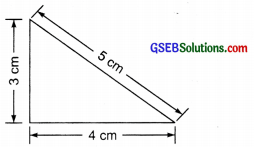(b) Length of each side = 9 cm
∵ The perimeter of an equilateral triangle
= 3 × length of one side
∴ The perimeter of the given equilateral triangle
= 3 × 9 cm = 27 cm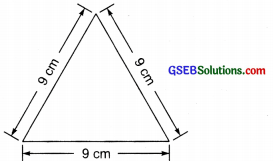(c) Sides of the given triangle are: 8 cm, 6 cm and 8 cm
∴ Perimeter of the triangle = sum of the sides
= 8 cm + 6 cm + 8 cm = 22 cm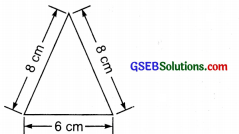Question 7.
Find the perimeter of a triangle with sides measuring 10 cm, 14 cm, and 15 cm.
Solution:
Sides of the triangle are: 10 cm, 14 cm and 15 cm
∴ Perimeter = sum of the sides
= 10 cm + 14 cm + 15 cm = 39 cmQuestion 8.
Find the perimeter of a regular hexagon with each side measuring 8 m.
Solution:
∵ The perimeter of a regular hexagon = 6 × one side of the hexagon
∴ Perimeter of the given regular hexagon = 6 × 8 m = 48 mQuestion 9.
Find the side of the square whose perimeter is 20 m.
Solution:
∵ The perimeter of the square = 20 m.
∵ Perimeter of square = 4 × length of one side
∴ 4 x Length of a side = 20 m
or Length of a side = $$\frac { 20 m }{ 2 }$$ = 5 mQuestion 10.
The perimeter of a regular pentagon is 100 cm. How long is each side?
Solution:
Perimeter of a regular pentagon = 100 cm
∵ Perimeter = 5 × Length of one side
∴ 5 × Length of one side = 100 cm
∴ Length of one side = $$\frac{100 \mathrm{~cm}}{5}$$ = 20 cmQuestion 11.
A piece of string is 30 cm long. What will be the length of each side of the string is used to form:
(a) a square?
(b) an equilateral triangle?
(c) a regular hexagon?
Solution:
Length of the given string = 30 cm
(a) ∵ The string is in the form of a square and perimeter of a square = 4 × Side
∴ side = $$\frac{30 \mathrm{~cm}}{4}$$ = 7.5 cm
∴ length of each side = 7.5 cm

(b) ∵ The string is in the form of an equilateral triangle
∴ perimeter = 3 × length of one side
or 3 × length of one side = 30 cm
or length of one side = $$\frac{30 \mathrm{~cm}}{3}$$ = 10 cm

(c) ∵ String is in the form of a regular hexagon and perimeter of a regular hexagon
= 6 × length of one side
6 × Length of one side = 30 cm
or Length of one side =$$\frac{30 \mathrm{~cm}}{6}$$= 5 cmQuestion 12.
Two sides of a triangle are 12 cm and 14 cm. The perimeter of the triangle is 36 cm. What is its third side?
Solution:
Perimeter of the triangle = 90 sum of its sides
= 12 cm + 14 cm + third side
= 26 cm + third side
But perimeter = 36 cm
∴ 26 cm + third side = 36 cm
or third side = 36 – 26 = 10 cm
Hence, the length of the third side of the triangle = 10 cmQuestion 13.
Find the cost of fencing a square park of side 250 m at the rate of 20 per meter.
Solution:
Side of the park = 250 m
∵ The park is in the form of a square.
∴ Perimeter = 4 × length of one side = 4 × 250 m = 1000 m
∵ Rate of fencing = ₹ 20 per meter
∴ Cost of fencing = ₹ 20 × 1000 = ₹ 20,000Question 14.
Find the cost of fencing a rectangular park of length 175 m and breadth 125 m at the rate of 12 per meter.
Solution:
Length of the park = 175 m
The breadth of the park = 125 m
Park is in the form of a rectangle.
Perimeter of a rectangle
= 2 × [length + breadth]
∴ Perimeter of the given park = 2 × [175 m + 125 m]
= 2 × 300 m = 600 m
Rate of fencing = ₹ 12 per metre
∴ Cost of fencing = ₹ 12 × 600 = ₹ 7200Question 15.
Sweety runs around a square park of side 75 m. Bulbul runs around a rectangular park with a length of 60 m and a breadth of 45 m. Who covers less distance?
Solution:
Side of the square park = 75 m
∴ Perimeter of the square park = 4 × side = 4 × 75 m = 300 m
i.e. Distance covered by Sweety = 300 m
Length of the rectangular park = 60 m
Breadth of the rectangular park = 45 m
∴ Perimeter of the rectangular park
= 2(length + breadth) = 2(60 m + 45 m)
= 2(105 m) = 210 m
i.e. Distance covered by Bulbul = 210 m
Since 210 < 300,
Thus, Bulbul covers less distance.Question 16.
What is the perimeter of each of the following figures? What do you infer from the answers?Solution:
(a) Length of a side of the square = 25 cm
∴ Perimeter = 4 × side = 4 × 25 = 100 cm

(b) Length of the rectangle = 40 cm
Breadth of the rectangle = 10 cm
∴ Perimeter = 2(length + breadth)
= 2(40 + 10) cm = 2 × (50 cm) = 100 cm

(c) Length of the rectangle = 30 cm
Breadth of the rectangle = 20 cm
∴ Perimeter = 2 (length + breadth)
= 2(30 cm + 20 cm) = 2 × 50 cm = 100 cm

(d) Sides of the given triangle are: 30 cm, 40 cm, and 30 cm
∴ Perimeter of the triangle = sum of the sides = 30 cm + 40 cm + 30 cm = 100 cm
Here, we observe that the perimeter of each figure is 100 cm or they have an equal perimeter.Question 17.
Avneet buys 9 square paving slabs, each with a side of $$\frac { 1 }{ 2 }$$ m. He lays them in the form of a square.
(a) What is the perimeter of his arrangement [Fig. (i)]?
(b) Shari does not like his arrangement. She gets him to lay them out like a cross. What is the perimeter of her arrangement [(Fig.(ii)]?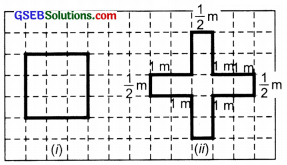(c) Which has a greater perimeter?
(d) Avneet wonders if there is a way of getting an even greater perimeter. Can you find a way of doing this? (The paving slabs must meet along complete edges i.e. they cannot be broken.)
Solution:
(a) The arrangement is in the form of a square of side∴ The perimeter of the square arrangement = 4 × side(b) Perimeter of the cross-arrangement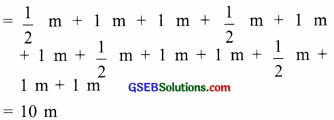(c) ∵ 10 m > 6 m
∴ Cross-arrangement has a greater perimeter.
(d) ∵ Total number of tiles = 9
∴ The following arrangement will also have a greater perimeter.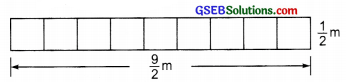Since, this arrangement is in the form of rectangle with length and breadth as $$\frac { 9 }{ 2 }$$ m and $$\frac { 1 }{ 2 }$$m respectively.
∴ Perimeter =